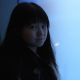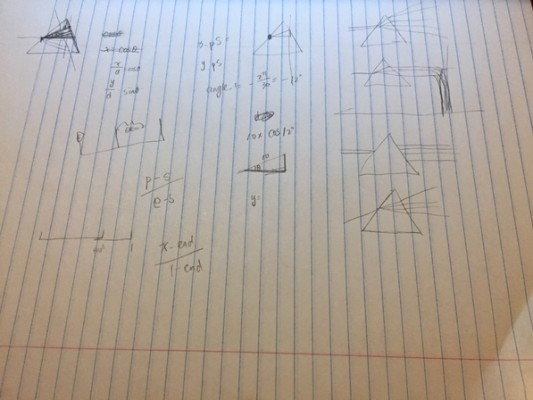# chen

## 09 Feb 2015What I did is based on Pink Floyd’s album cover — Dark Side of the Moon.At first, I animated what I saw on this album cover, and here is the scratch. (I should say I’m really not good at scratching.)Then I thought it might be more reasonable to animate it as cannon fire.

So here is the final animation:I think I do make the original image more vivid, but it still looks not consistent. It’ll be better to change the circles into something like bubbles, or something more interesting.

```// Based on Prof. Golan Levin's template.

//===================================================
// Global variables.

int nFramesInLoop = 100; // For lenticular export, REMEMBER TO CHANGE THIS to 10!
int nElapsedFrames;
boolean bRecording;

//===================================================
void setup() {
size (500, 500);
bRecording = false;
nElapsedFrames = 0;
frameRate (nFramesInLoop);
}
//===================================================
void keyPressed() {
// Press a key to export frames to the output folder
bRecording = true;
nElapsedFrames = 0;
}

//===================================================
void draw() {

// Compute a percentage (0...1) representing where we are in the loop.
float percentCompleteFraction = 0;
if (bRecording) {
percentCompleteFraction = (float) nElapsedFrames / (float)nFramesInLoop;
}
else {
float modFrame = (float) (frameCount % nFramesInLoop);
percentCompleteFraction = modFrame / (float)nFramesInLoop;
}

// Render the design, based on that percentage.
renderMyDesign (percentCompleteFraction);

// If we're recording the output, save the frame to a file.
if (bRecording) {
String  myName = "chenlian";
saveFrame("output/"+ myName + "-loop-" + nf(nElapsedFrames, 4) + ".png");
nElapsedFrames++;
if (nElapsedFrames == nFramesInLoop) {
bRecording = false;
}
}
}

//===================================================
void renderMyDesign (float percent) {

// This is an example of a function that renders a temporally looping design.
// It takes a "percent", between 0 and 1, indicating where we are in the loop.
// This example uses two different graphical techniques.
// Use or delete whatever you prefer from this example.
// Remember to SKETCH FIRST!

//----------------------
// here, I set the background and some other graphical properties
background (34,31,22);

//stroke (100, 0, 0);
//strokeWeight (6);

//----------------------
// Here, I'll write name on the screen.

textSize(20);
fill(0, 102, 153, 51);
text("Chen Liang", 60, 40);

//----------------------
// Here, I draw incident light.
float x_pointS = 0;  // the start point of incident light
float y_pointS = height/1.8;
float x_pointE = 0;  // the start point of incident light
float y_pointE = height/1.8;

float finalPercent = 0.19;
if (percent < finalPercent) {     x_pointE  = percent * width;     y_pointE = y_pointS - (height/1.8-height/2.4)/0.401 * percent;   } else {     x_pointE  = width*0.401;     y_pointE = height/2.4;   }      strokeWeight(9);   line    (x_pointS, y_pointS, x_pointE, y_pointE);      //----------------------   // Here, I draw five colors inside the glass   float x_pointStart = width*0.401;   float y_pointStart = height/2.4;      float angle_1 = -TWO_PI/25;   float angle_2 = -TWO_PI/40;   float angle_3 = -TWO_PI/90;   float angle_4 = TWO_PI/150;   float angle_5 = TWO_PI/50;      float startPercent = 0.19;   float endPercent = 0.33;   float interval = endPercent - startPercent;      float distance_1 = width/5.8;   float distance_2 = width/5.5;   float distance_3 = width/5.3;   float distance_4 = width/5;   float distance_5 = width/4.8;      float x_point_1;   float y_point_1;   float x_point_2;   float y_point_2;   float x_point_3;   float y_point_3;   float x_point_4;   float y_point_4;   float x_point_5;   float y_point_5;      float x_endpoint_1 = distance_1 * cos(angle_1) + x_pointStart;   float y_endpoint_1 = y_pointStart + distance_1 * sin(angle_1);   float x_endpoint_2 = distance_2 * cos(angle_2) + x_pointStart;   float y_endpoint_2 = y_pointStart + distance_2 * sin(angle_2);   float x_endpoint_3 = distance_3 * cos(angle_3) + x_pointStart;   float y_endpoint_3 = y_pointStart + distance_3 * sin(angle_3);   float x_endpoint_4 = distance_4 * cos(angle_4) + x_pointStart;   float y_endpoint_4 = y_pointStart + distance_4 * sin(angle_4);   float x_endpoint_5 = distance_5 * cos(angle_5) + x_pointStart;   float y_endpoint_5 = y_pointStart + distance_5 * sin(angle_5);      noStroke();      float x = (percent - endPercent)/(1-endPercent);   float a = 0.793;   float b = 1;   float om2a = 1.0 - 2.0*a;   float t = (sqrt(a*a + om2a*x) - a)/om2a;   float percentage = (1.0-2.0*b)*(t*t) + (2*b)*t;      float emitter_distance = width/2;      float emitter_angle_1 = (angle_1 + angle_2)/2;   float emitter_angle_2 = (angle_3 + angle_2)/2;   float emitter_angle_3 = (angle_3 + angle_4)/2;   float emitter_angle_4 = (angle_5 + angle_4)/2;   float emitter_x_1 = x_endpoint_1 + emitter_distance * cos(emitter_angle_1) * percentage;   float emitter_y_1 = y_endpoint_1 + emitter_distance * sin(emitter_angle_1) * percentage;   float emitter_x_2 = x_endpoint_2 + emitter_distance * cos(emitter_angle_2) * percentage;   float emitter_y_2 = y_endpoint_2 + emitter_distance * sin(emitter_angle_2) * percentage;   float emitter_x_3 = x_endpoint_3 + emitter_distance * cos(emitter_angle_3) * percentage;   float emitter_y_3 = y_endpoint_3 + emitter_distance * sin(emitter_angle_3) * percentage;   float emitter_x_4 = x_endpoint_4 + emitter_distance * cos(emitter_angle_4) * percentage;   float emitter_y_4 = y_endpoint_4 + emitter_distance * sin(emitter_angle_4) * percentage;      float amplitude = 20;   float radius = percentage * amplitude + width/60;   if (percent > endPercent) {
fill (215,101,8);
fill (233,209,9);
fill(117,141,3);
fill(23,160,152);
}

if (percent >= startPercent && percent < = endPercent){     x_point_1 = distance_1 * cos(angle_1) * (percent - startPercent)/interval + x_pointStart;     y_point_1 = y_pointStart + distance_1 * sin(angle_1) * (percent - startPercent)/interval;     x_point_2 = distance_2 * cos(angle_2) * (percent - startPercent)/interval + x_pointStart;     y_point_2 = y_pointStart + distance_2 * sin(angle_2) * (percent - startPercent)/interval;     x_point_3 = distance_3 * cos(angle_3) * (percent - startPercent)/interval + x_pointStart;     y_point_3 = y_pointStart + distance_3 * sin(angle_3) * (percent - startPercent)/interval;     x_point_4 = distance_4 * cos(angle_4) * (percent - startPercent)/interval + x_pointStart;     y_point_4 = y_pointStart + distance_4 * sin(angle_4) * (percent - startPercent)/interval;     x_point_5 = distance_5 * cos(angle_5) * (percent - startPercent)/interval + x_pointStart;     y_point_5 = y_pointStart + distance_5 * sin(angle_5) * (percent - startPercent)/interval;          fill (215,101,8);     triangle(x_pointStart, y_pointStart, x_point_1, y_point_1,x_point_2, y_point_2);     fill (233,209,9);     triangle(x_pointStart, y_pointStart, x_point_3, y_point_3,x_point_2, y_point_2);     fill(117,141,3);     triangle(x_pointStart, y_pointStart, x_point_3, y_point_3,x_point_4, y_point_4);     fill(23,160,152);     triangle(x_pointStart, y_pointStart, x_point_5, y_point_5,x_point_4, y_point_4);       } else if (percent > endPercent){
x_point_1 = distance_1 * cos(angle_1) + x_pointStart;
y_point_1 = y_pointStart + distance_1 * sin(angle_1);
x_point_2 = distance_2 * cos(angle_2) + x_pointStart;
y_point_2 = y_pointStart + distance_2 * sin(angle_2);
x_point_3 = distance_3 * cos(angle_3) + x_pointStart;
y_point_3 = y_pointStart + distance_3 * sin(angle_3);
x_point_4 = distance_4 * cos(angle_4) + x_pointStart;
y_point_4 = y_pointStart + distance_4 * sin(angle_4);
x_point_5 = distance_5 * cos(angle_5) + x_pointStart;
y_point_5 = y_pointStart + distance_5 * sin(angle_5);

fill (215,101,8);
triangle(x_pointStart, y_pointStart, x_point_1, y_point_1,x_point_2, y_point_2);
fill (233,209,9);
triangle(x_pointStart, y_pointStart, x_point_3, y_point_3,x_point_2, y_point_2);
fill(117,141,3);
triangle(x_pointStart, y_pointStart, x_point_3, y_point_3,x_point_4, y_point_4);
fill(23,160,152);
triangle(x_pointStart, y_pointStart, x_point_5, y_point_5,x_point_4, y_point_4);
}

//----------------------
// Here, I calculate the three points of triangle.
float cx = width/2;
float cy = height/2;
float d  = width/4;

float x_pointU = cx;  // the upper point of triangle
float y_pointU = cy - d;
float x_pointL = cx - d/2*1.7;  // the left point of triangle
float y_pointL = cy + d/2;
float x_pointR = cx + d/2*1.7;// the right point of triangle
float y_pointR = cy + d/2;

stroke (229,208,154);
strokeWeight(5);
line(x_pointU,y_pointU,x_pointL,y_pointL);
line(x_pointR,y_pointR,x_pointL,y_pointL);
line(x_pointU,y_pointU,x_pointR,y_pointR);
}```